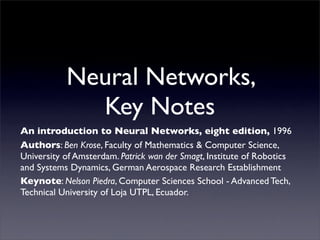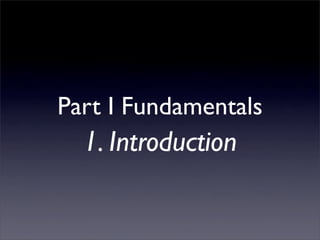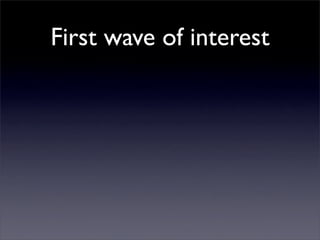Successfully reported this slideshow.

# Fundamental, An Introduction to Neural Networks

70

Share×
1 of 85
1 of 85

# Fundamental, An Introduction to Neural Networks

70

Share

An introduction to Neural Networks, eight edition, 1996
Authors: Ben Krose, Faculty of Mathematics & Computer Science, University of Amsterdam. Patrick wan der Smagt, Institute of Robotics and Systems Dynamics, German Aerospace Research Establishment
Keynote: Nelson Piedra, Computer Sciences School - Advanced Tech, Technical University of Loja UTPL, Ecuador.

An introduction to Neural Networks, eight edition, 1996
Authors: Ben Krose, Faculty of Mathematics & Computer Science, University of Amsterdam. Patrick wan der Smagt, Institute of Robotics and Systems Dynamics, German Aerospace Research Establishment
Keynote: Nelson Piedra, Computer Sciences School - Advanced Tech, Technical University of Loja UTPL, Ecuador.

## More Related Content

### Related Books

Free with a 14 day trial from Scribd

See all

### Related Audiobooks

Free with a 14 day trial from Scribd

See all

### Fundamental, An Introduction to Neural Networks

1. 1. Neural Networks, Key Notes An introduction to Neural Networks, eight edition, 1996 Authors: Ben Krose, Faculty of Mathematics & Computer Science, University of Amsterdam. Patrick wan der Smagt, Institute of Robotics and Systems Dynamics, German Aerospace Research Establishment Keynote: Nelson Piedra, Computer Sciences School - Advanced Tech, Technical University of Loja UTPL, Ecuador.
2. 2. Part I Fundamentals 1. Introduction
3. 3. First wave of interest
4. 4. First wave of interest • First wave of interest emerged after the introduction of simpliﬁed neurons by McCullock and Pitts in 1943.
5. 5. First wave of interest • First wave of interest emerged after the introduction of simpliﬁed neurons by McCullock and Pitts in 1943. • These neurons were introduced as models of biological neurons and as conceptual components for circuts that could perform computational tasks.
6. 6. ANN, “black age”
7. 7. ANN, “black age” • Perceptrons book (Minsky & Papert, 1969): showed deﬁciencies of perceptrons models, most neural network funding was redirected and researches left the ﬁeld
8. 8. ANN, “black age” • Perceptrons book (Minsky & Papert, 1969): showed deﬁciencies of perceptrons models, most neural network funding was redirected and researches left the ﬁeld • Only a few researchers continued their efforts, most notably Teuvo Kohoen, Stephen Grossberg, James Anderson, and Kunihiko Fukushima
9. 9. ANN re-emerged
10. 10. ANN re-emerged • Early eighties: ANN, re-emerged only after some important theorical results, most notably the discovery of error back- propagation, and new hardware developments increased the processing capacities.
11. 11. ANN re-emerged • Early eighties: ANN, re-emerged only after some important theorical results, most notably the discovery of error back- propagation, and new hardware developments increased the processing capacities. • Nowdays most universities have a neural networks groups (i.e. Advanced Tech - UTPL)
12. 12. ¿How be can adequality characterised A.N.N.?
13. 13. ¿How be can adequality characterised A.N.N.? • Artiﬁcial neural networks can be most adequately characterised as “computational models” with particular properties such as the ability,
14. 14. ¿How be can adequality characterised A.N.N.? • Artiﬁcial neural networks can be most adequately characterised as “computational models” with particular properties such as the ability, • to adapt or learn,
15. 15. ¿How be can adequality characterised A.N.N.? • Artiﬁcial neural networks can be most adequately characterised as “computational models” with particular properties such as the ability, • to adapt or learn, • to generalise, or
16. 16. ¿How be can adequality characterised A.N.N.? • Artiﬁcial neural networks can be most adequately characterised as “computational models” with particular properties such as the ability, • to adapt or learn, • to generalise, or • to cluster or organise data, and
17. 17. ¿How be can adequality characterised A.N.N.? • Artiﬁcial neural networks can be most adequately characterised as “computational models” with particular properties such as the ability, • to adapt or learn, • to generalise, or • to cluster or organise data, and • which operation is based on parallel processing.
18. 18. ¿How be can adequality characterised A.N.N.? • Artiﬁcial neural networks can be most adequately characterised as “computational models” with particular properties such as the ability, • to adapt or learn, • to generalise, or • to cluster or organise data, and • which operation is based on parallel processing. • Also exist parallels with biological systems
20. 20. to to adapt learn
21. 21. to to adapt learn parallel process
22. 22. to to adapt learn to parallel organise process data
23. 23. to to to cluster adapt learn to parallel organise process data
24. 24. to to to cluster adapt learn to parallel organise process data Above slide shows properties can be attributed to neural network models and existing (non-neural) models
25. 25. Extent the neural approach proves to be better suited for certain applications than existing models
26. 26. Part I Fundamentals 2. Fundamentals
27. 27. A framework for distributed representation
28. 28. A framework for distributed representation • To understand ANN, thinking on the parallel distributed processing (PDP) idea
29. 29. A framework for distributed representation • To understand ANN, thinking on the parallel distributed processing (PDP) idea • An artiﬁtial network consists of a pool of simple processing units wich comunicate by sending signals to each other over a large number of weighted connections.
30. 30. • 1/2 Rumelhart and McClelland, 1986:
31. 31. • 1/2 Rumelhart and McClelland, 1986: • a set o processing units (‘neurons’, ‘cells’);
32. 32. • 1/2 Rumelhart and McClelland, 1986: • a set o processing units (‘neurons’, ‘cells’); • a state o activation y for every unit, wich k equivalent to the output of the unit;
33. 33. • 1/2 Rumelhart and McClelland, 1986: • a set o processing units (‘neurons’, ‘cells’); • a state o activation y for every unit, wich k equivalent to the output of the unit; • connections between the units. Generally each conection is deﬁned by a weight wjk wich determines the effect wich the signal of unit j has on unit k;
34. 34. • 1/2 Rumelhart and McClelland, 1986: • a set o processing units (‘neurons’, ‘cells’); • a state o activation y for every unit, wich k equivalent to the output of the unit; • connections between the units. Generally each conection is deﬁned by a weight wjk wich determines the effect wich the signal of unit j has on unit k; • a propagation rule, wich determines the effective input sk of a unit from its external inputs.
35. 35. • 2/2 Rumelhart and McClelland, 1986:
36. 36. • 2/2 Rumelhart and McClelland, 1986: • an activation function F , wich determines k the new level of activation based on the effective input sk(t) and the current activation yk(t);
37. 37. • 2/2 Rumelhart and McClelland, 1986: • an activation function F , wich determines k the new level of activation based on the effective input sk(t) and the current activation yk(t); • an external input (aka bias, offset) θk for each unit;
38. 38. • 2/2 Rumelhart and McClelland, 1986: • an activation function F , wich determines k the new level of activation based on the effective input sk(t) and the current activation yk(t); • an external input (aka bias, offset) θk for each unit; • a method for information gathering (the learning rule);
39. 39. • 2/2 Rumelhart and McClelland, 1986: • an activation function F , wich determines k the new level of activation based on the effective input sk(t) and the current activation yk(t); • an external input (aka bias, offset) θk for each unit; • a method for information gathering (the learning rule); • an environment within wich the system must operate, provinding input signals and -if necesary- error signals
40. 40. Processing Units
41. 41. Processing Units • Each unit performs a relatively simple job:
42. 42. Processing Units • Each unit performs a relatively simple job: • a) receive input from neighbours or external sources an use this to compute an output which is propagated to other units;
43. 43. Processing Units • Each unit performs a relatively simple job: • a) receive input from neighbours or external sources an use this to compute an output which is propagated to other units; • b) adjustment of the weights
44. 44. Processing Units • Each unit performs a relatively simple job: • a) receive input from neighbours or external sources an use this to compute an output which is propagated to other units; • b) adjustment of the weights • The system is inherently parallel in the sense that many units can carry out their computations at the same time
45. 45. k w1k yk w2k fk sk = Σj wjk yj + θk wjk wnk j y θk The basic components of an artificial neural network. The propagation rule used here is the standard wighted summation
46. 46. Thre types of units input units, i: which receive data from outside the neural network output units, o: which send data out of neural network hidden units, h: whose input and output signals remain within the neural network
47. 47. update of units Synchronously: all units update their activation simultanously Asynchronously: each unit has a (usually ﬁxed) probability of updating its activation at a time t, and usually only one unit will be to do this at a time; in some cases the latter model has some advantages
48. 48. Conections between units sk (t) = Σ wjk (t) yj (t)+ θk j
49. 49. Conections between units • Assume that unit provides an additive contribution to the input of the unit which it is connected sk (t) = Σ wjk (t) yj (t)+ θk j
50. 50. Conections between units • Assume that unit provides an additive contribution to the input of the unit which it is connected • The total input to unit k is simply the weighted sum of the separate outputs from each of the connected units plus a bias or offset term θk sk (t) = Σ wjk (t) yj (t)+ θk j
51. 51. Conections between units • Assume that unit provides an additive contribution to the input of the unit which it is connected • The total input to unit k is simply the weighted sum of the separate outputs from each of the connected units plus a bias or offset term θk • A positive w is considerad excitation and jk negative wjk as inhibition. sk (t) = Σ wjk (t) yj (t)+ θk j
52. 52. Conections between units • Assume that unit provides an additive contribution to the input of the unit which it is connected • The total input to unit k is simply the weighted sum of the separate outputs from each of the connected units plus a bias or offset term θk • A positive w is considerad excitation and jk negative wjk as inhibition. • The units of propagation rule be call sigma units sk (t) = Σ wjk (t) yj (t)+ θk j
53. 53. Different propagation rule sk (t) = Σ wjk (t) ∏ yjm (t)+ θk (t) j m
54. 54. Different propagation rule • Propagation rule for the sigma - Pi unit, Feldman and Ballard, 1982. sk (t) = Σ wjk (t) ∏ yjm (t)+ θk (t) j m
55. 55. Different propagation rule • Propagation rule for the sigma - Pi unit, Feldman and Ballard, 1982. • Often, the yjm are weighted before multiplication. Although these units are not frequently used, they their value for gating of input, as well as implementation of lookup tables (Mel 1990) sk (t) = Σ wjk (t) ∏ yjm (t)+ θk (t) j m
56. 56. Activation and output rules • New value de activation: we need a function fk which takes the total input sk (t) and the current activation yk (t) and produced a new value of the activation of the unit k. yk (t+1) = fk(yk (t) , sk (t) )
57. 57. • Often, the activation function is a nondecreasing function of the total input of the unit yk (t+1) = fk( sk (t) ) = fk( Σ wjk (t) yj (t)+ θk (t) ) j Sgn sigmoid i i i semi linear hard limiting linear o semi linear smoothly limiting threshold function function threshold
58. 58. • For this smoonthly limiting function often a sigmoid (S-shaped) function like: yk = fk( sk )=1 / ( 1 +e-sk ) • In some cases, the output of a unit can be a stochastic function of the total input of the unit. In that case the activation is not deterministically determined by the neuron input, but the neuron input determines the probability p that a neuron get a high activation rule p( yk ← 1 ) = 1/ ( 1 +e-sk /T )
59. 59. Network topologies • This section focuses on the pattern of connections between the units and the propagation of data: • Feed - forward networks • Recurrent networks that do contain feedback connections
60. 60. Feed-forward networks • The data processing can extend over multiple (layers of) units, but no feedback connections are present, that is, connections extending from outputs of units to input of units in the same layer or previous layers
61. 61. Recurrent networks that do contain feedback connections
62. 62. Recurrent networks that do contain feedback connections • Contrary to feed-forward networks, the dynamical properties of the network are important.
63. 63. Recurrent networks that do contain feedback connections • Contrary to feed-forward networks, the dynamical properties of the network are important. • In some cases, the activation values of the units under go a relaxation process such that the network will evolve to a stable state in wich these activations do not change anymore.
64. 64. Recurrent networks that do contain feedback connections • Contrary to feed-forward networks, the dynamical properties of the network are important. • In some cases, the activation values of the units under go a relaxation process such that the network will evolve to a stable state in wich these activations do not change anymore. • In other applications, the change of the activation values of the output neurons are signiﬁcant, such that the dynamical behaviour constitutes the output of the network (Pearlmutter, 1990)
65. 65. Recurrent networks that do contain feedback connections • Contrary to feed-forward networks, the dynamical properties of the network are important. • In some cases, the activation values of the units under go a relaxation process such that the network will evolve to a stable state in wich these activations do not change anymore. • In other applications, the change of the activation values of the output neurons are signiﬁcant, such that the dynamical behaviour constitutes the output of the network (Pearlmutter, 1990) • Classical examples of feed-forward networks are the Perceptron and Adaline.
66. 66. Training of artiﬁcial neural networks
67. 67. Training of artiﬁcial neural networks • A neural network has to be conﬁgured such that the application of a set of inputs produces (either ‘direct’ or via a relaxation process) the desired set ot output.
68. 68. Training of artiﬁcial neural networks • A neural network has to be conﬁgured such that the application of a set of inputs produces (either ‘direct’ or via a relaxation process) the desired set ot output. • One way is to set the weights explicity, using a priori knowledge.
69. 69. Training of artiﬁcial neural networks • A neural network has to be conﬁgured such that the application of a set of inputs produces (either ‘direct’ or via a relaxation process) the desired set ot output. • One way is to set the weights explicity, using a priori knowledge. • Other way is to ‘train’ the neural network by feeding it teaching patterns and letting it change its weights according to some learning rule.